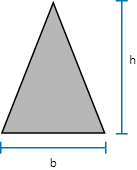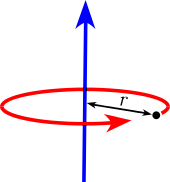•Sign In
• Hire UsUh Oh! It seems you’re using an Ad blocker!

We always struggled to serve you with the best online calculations, thus, there's a humble request to either disable the AD blocker or go with premium plans to use the AD-Free version for calculators.

Or# Moment of Inertia Calculator

To SelectBase Width (b)

D(Outer Diameter)

d(Inner Diameter)

Height (h)

Distance to height(a)

TFw

TFt

BFw

BFt

LFt

LFh

Wh

Wt

r

b1

h

Units

Table of Content

 1 What is priceeight Class? 2 priceeight Class Chart: 3 How to Calculate priceeight Density (Step by Step): 4 Factors that Determine priceeight Classification: 5 What is the purpose of priceeight Class? 6 Are mentioned priceeight Classes verified by the officials? 7 Are priceeight Classes of UPS and FedEx same?

Get the Widget!

Add this calculator to your site and lets users to perform easy calculations.

Feedback

How easy was it to use our calculator? Did you face any problem, tell us!

An online moment of inertia calculator is exclusively programmed to determine the moment of inertia of common geometrical figures like triangle, rectangle, and many more. You can not only determine this particular quantity, but also area, centroid of beam, and section modulus by using this free calculator.

If you are interested to know more about the moment of inertia, go through the context below for a better understanding.

Keep Scrolling!

## What Is The Moment Of Inertia?

In the context of physical sciences:

“A specific quantity that is responsible for producing the torque in a body about a rotational axis is called the moment of inertia”First Moment Of Inertia:

“It represents the spatial distribution of the given shape in relation to its relative axis”

Second Moment Of Inertia:

“This specific property displays the point distribution with respect to the axis”

### Moment Of Inertia Formula:

$$I = \frac{L}{𝓌}$$

Where:
L = Angular Momentum
𝓌 = Angular Frequency
I = Inertia

#### More Formulas:

Finding moments of inertia may involve lots of complex calculations that are not easy to resolve every time. But here we will be using formulas for some of the basic shapes. Keep one thing in mind that these formulas hold only when the x and y axis of the given figure passes from the centroid.

The formulas are as follows:

Moment Of Inertia Of Triangle:

$$I_{x} = \frac{\left(width\right) * \left(height\right)^{3}}{36}$$
$$I_{y} = \frac{\left(\left(height\right) * \left(width\right)^{3} – \left(height\right) * a * \left(width\right)^{2} + \left(width\right) * \left(height\right) * \left(a\right)^{2}\right)}{36}$$

Moment Of Inertia Rectangle:

$$I_{x} = \frac{\left(width\right) * \left(height\right)^{3}}{12}$$
$$I_{x} = \frac{\left(height\right) * \left(width\right)^{3}}{12}$$

Moment Of Inertia Of Hollow Rectangle:

$$I_{x} = \frac{b * \left(h\right)^{3} – b_{1} * \left(h_{1}^{3}\right)}{12}$$
$$I_{y} = \frac{\left(b\right)^3 * h – b_{1}^3 * h_{1}}{12}$$

In case of any hurdle, try using the area moment of inertia calculator for accurate and instant outputs of various parameters that are related to the moment of inertia.

Moment Of Inertia For Circle:

$$I_{x} = I_{y} = \frac{\pi}{4} * \left(radius\right)^{4}$$

Moment Of Inertia Of Hollow Circle:

$$I_{x} = I_{y} = \frac{\pi}{4} \left(r_{2}^4 – r_{1}^4\right)$$

Moment Of Inertia Of Semicircle:

$$I_{x} = [\frac{\pi}{8} – \frac{8}{\left(9 * \pi\right)}] * \left(radius\right)^{4}$$
$$I_{y} = \frac{\pi}{8} * \left(radius\right)^{4}$$

Moment Of Inertia Of Ellipse:

$$I_{x} = \frac{\pi}{4} * \left(radius\right)_{x} * \left(radius\right)_{y}^{3}$$
$$I_{y} = \frac{\pi}{4} * \left(radius\right)_{y} * \left(radius\right)_x^{3}$$

Moment Of Inertia Of Regular Hexagon:

$$I_{x} = I_{y} = 5 * \sqrt{\frac{\left(3\right)}{16}} * \left(side length\right)^{4}  Moment Of Inertia Of I Beam:$$ I_{x} = \frac{H^{3} * b}{12} + 2[\frac{h^{3} * B}{12} + h * B * \frac{\left(H + h\right)^{2}}{4}]  I_{y} = \frac{b^{3} * H}{12} + 2 * \left(\frac{B^{3} * h}{12}\right) $$Moment Of Inertia Of T Beam:$$ I_{x} = \frac{TFw * TFt^{3}}{12} + \frac{Wt * Wh^{3}}{12} + TFw * TFt\left(Wh + \frac{TFt}{2} – y_{bot}\right)^{2} + Wt * Wh * \left(\frac{Wh}{2} – y_{bot}\right)^{2}  I_{y} = \frac{TFt * TFw^{3}}{12} + \frac{Wh * Wt^{3}}{12} $$Moment Of Inertia Of L Beam: Moment Of Inertia Of Channel:$$ I_{x} = \frac{TFw * TFt^{3}}{12} + \frac{BFw * BFt^{3}}{12} + \frac{Wt * h}{12} + TFw * TFt * \left(h – \frac{TFt}{2} – y_{bot}\right)^{2} + BFw * BFt * \left(\frac{Bft}{2} – y_{bot}\right) ^{2} + Wt * h * \left(\frac{h}{2} – y_{bot}\right)^{2}  I_{y} = \frac{TFt * TFw^{3}}{12} + \frac{BFt * BFw^{3}}{12} + \frac{h * Wt^{3}}{12} +TFt * TFw * \left(Wt + \frac{TFw}{2} – x_{left}\right)^{2} + BFt * BFw * \left(Wt +\frac{BFw}{2} – x_{left}\right)^{2} + h * Wt * \left(\frac{Wt}{2} – x_{left}\right)^{2} $$As there are many terms involved in these formulas, the free moment of inertia calculator takes a couple of seconds in resolving them and displaying answers. ### How To Calculate Moment Of Inertia? Here we will be solving a couple of examples related to inertial moments. Stay with it! Example # 01: Calculate moment of inertia of an object revolving with angular acceleration of $$2\frac{rad}{s^{2}}$$ with angular torque of about 3Nm. Determine its moment of inertia. Solution: We know that:$$ I = \frac{L}{𝓌}  I = \frac{3}{2}  I = 1.5kgm^{2} $$Example # 02: Determine the moment of inertia of the circle in terms of its polar coordinates having a radius of 4cm. Solution: As we know that:$$ I_{x} = I_{y} = \frac{\pi}{4} * \left(radius\right)^{4}  I_{x} = I_{y} = \frac{3.14}{4} * \left(4\right)^{4}  I_{x} = I_{y} = 0.785 * 256  I_{x} = I_{y} = 200.96kgm^{2} 

Here the free polar moment of inertia calculator also shows the same results but in a very short span of seconds, saving your precious time.

### How Moment Of Inertia Calculator Works?

Except manual calculations, make use of this second moment of area calculator that generates accurate outputs within a couple of clicks. Let’s find how!

Input:

• In the beginning, make a selection of the geometrical figure from the drop down menu for which you want to determine the moment of inertia
• After you make a selection, write down the values of the parameter against that selected figure along with the units
• Tap the calculate button

Output:

Depending upon the input provided, the moi calculator determines either:

• Moment of inertia about the x-axis and y-axis
• Total area of the given figure
• Centroid of the figure in terms of x and y coordinates
• Section modulus of the figure in terms of its coordinates

## FAQ’s:

### Why is Newton’s first law called inertia?

As the newton’s first law of motion states that:

“A body remains at rest or in continuous motion until or unless acted upon by external force to either move it or stop it”

The above statement defines the inertia of a particular body. This is why the 1st law of motion gives us the definition of inertia.

### What is Galileo’s law of inertia?

This law states that:

“A particular object remains in the state of motion if no net force acts upon it to stop it.

### What is inertia?

A particular property of the matter with the help of which it keeps itself either in the state of continuous motion or rest is known as the inertia.

### What does moment of inertia tell?

In actuality, the moment of inertia tells you how difficult it is to rotate a certain object about an axis that could easily be determined by using the free online rotational inertia calculator.

## Conclusion:

The moment of inertia is very important to keep the heavy objects in a smooth motion without any damage. The swings like skywheel, discovery, roller coaster, and many others are operated on the moment of inertia. That is why the free mass moment of inertia calculator helps you to determine the moment of inertia to avoid any hurdle before starting such huge swings.

From the source of Wikipedia: Moment of inertia, Simple pendulum, Compound pendulums, Motion in a fixed plane, Rigid body, Angular momentum, Kinetic energy, Resultant torque, Parallel axis theorem, Scalar moment of inertia in a plane, Inertia tensor, Alternate inertia convention

From the source of Khan Academy: Rotational inertia, More on moment of inertia, Rotational kinetic energy,  Moments

From the source of Lumen Learning: Rotational Kinetic Energy, Moment of Inertia, Rotational Energy